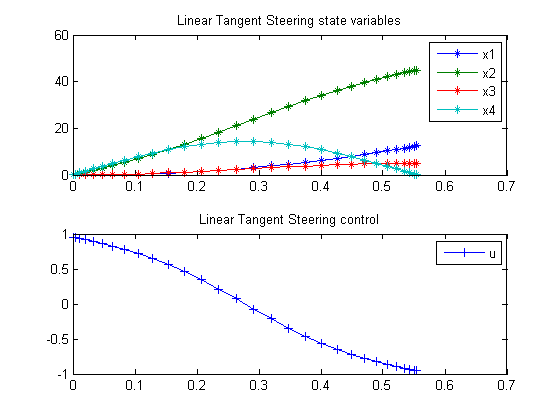57  Linear Tangent Steering Problem

Benchmarking Optimization Software with COPS Elizabeth D. Dolan and Jorge J. More ARGONNE NATIONAL LABORATORY

57.1  Problem Formulation

Find u(t) over t in [0; t_F ] to minimize

 J = tf

subject to:

 d2y1 dt2
= a*cos(u
 d2y2 dt2
= a*sin(u
|u| <=
 pi 2

 y1:2(0) = 0
 dy1:2 dt
= 0
 a = 1
 y2(f) = 5
 dy1:2 dt
(f) = [45  0]

The following transformation gives a new formulation:

 x1 = y1
x2 =
 dy1 dt

 x3 = y2
x4 =
 dy2 dt

 dx1 dt
= x2
 dx2 dt
= a*cos(u
 dx3 dt
= x4
 dx4 dt
= a*sin(u

Reference: 

57.2  Problem setup

toms t
toms t_f
p = tomPhase('p', t, 0, t_f, 30);
setPhase(p);

tomStates x1 x2 x3 x4
tomControls u

% Initial guess
x0 = {t_f == 1
icollocate({
x1 == 12*t/t_f
x2 == 45*t/t_f
x3 == 5*t/t_f
x4 == 0})};

% Box constraints
cbox = {sqrt(eps) <= t_f
-pi/2 <= collocate(u) <= pi/2};

% Boundary constraints
cbnd = {initial({x1 == 0; x2 == 0; x3 == 0; x4 == 0})
final({x2 == 45; x3 == 5; x4 == 0})};

% ODEs and path constraints
a = 100;
ceq = collocate({dot(x1) == x2
dot(x2) == a*cos(u)
dot(x3) == x4
dot(x4) == a*sin(u)});

% Objective
objective = t_f;

57.3  Solve the problem

options = struct;
options.name = 'Linear Tangent Steering';
options.solver = 'knitro';
solution = ezsolve(objective, {cbox, cbnd, ceq}, x0, options);
t  = subs(collocate(t),solution);
x1 = subs(collocate(x1),solution);
x2 = subs(collocate(x2),solution);
x3 = subs(collocate(x3),solution);
x4 = subs(collocate(x4),solution);
u  = subs(collocate(u),solution);
Problem type appears to be: lpcon
Starting numeric solver
===== * * * =================================================================== * * *
TOMLAB - Tomlab Optimization Inc. Development license  999001. Valid to 2011-02-05
=====================================================================================
Problem: ---  1: Linear Tangent Steering        f_k       0.554570876848855420
sum(|constr|)      0.000053410901111122
f(x_k) + sum(|constr|)      0.554624287749966530
f(x_0)      1.000000000000000000

Solver: KNITRO.  EXIT=0.  INFORM=0.
Default NLP KNITRO
Locally optimal solution found

FuncEv   14 GradEv  152 ConstrEv   13 ConJacEv  152 Iter   11 MinorIter   12
CPU time: 0.312500 sec. Elapsed time: 0.312000 sec.

57.4  Plot result

subplot(2,1,1)
plot(t,x1,'*-',t,x2,'*-',t,x3,'*-',t,x4,'*-');
legend('x1','x2','x3','x4');
title('Linear Tangent Steering state variables');

subplot(2,1,2)
plot(t,u,'+-');
legend('u');
title('Linear Tangent Steering control');